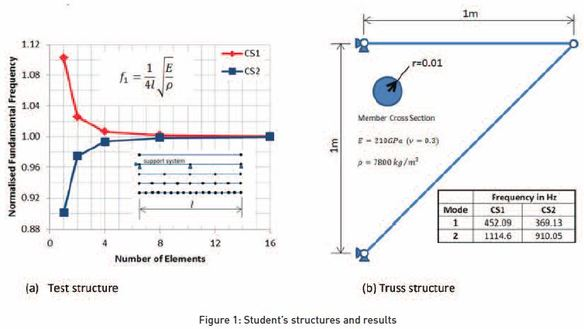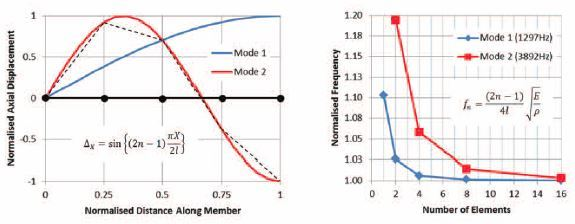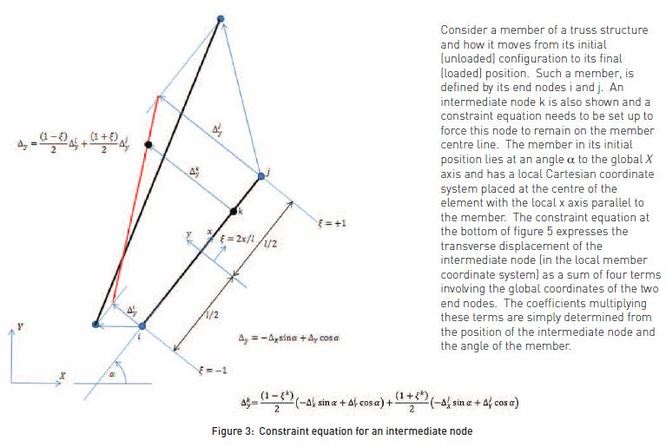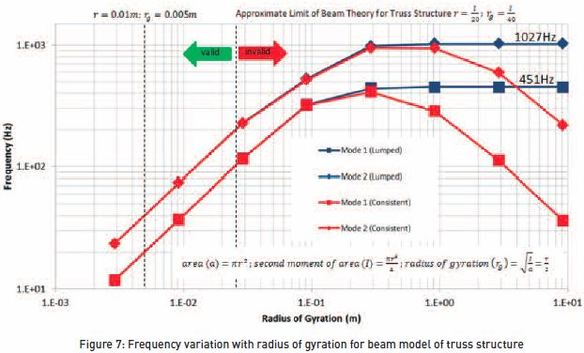Description Qty Item Price

sub total£0.00
proceed to checkout

## Solution - NAFEMS Benchmark Challenge 05

### July 24th 2016The Benchmark Challenge was launched the October 2014 edition of Benchmark and on the NAFEMS Challenge blog. The concept behind this initiative is to provide seemingly simply problems for readers to consider. The challenges will typically contain in interesting ‘twist’ which is intended to be thought-provoking and to provide an opportunity for learning.

challenge@nafems.org

## Raison d’être for the Challenge

This challenge raises an interesting question namely is the standard bar element, available in most if not all commercial finite element systems, able accurately to model the dynamic behaviour of truss structures when only a single element is used to represent each member? The test structure investigated by the student indicates that by using only a single element per member one standard bar element, available in most if not finite element systems, able accurately to model the dynamic behaviour of truss structures the dynamic behaviour of truss structures a single element is used to represent each member? The test structure investigated by the student by using only a single element per member one is likely to have some significant error in the finite element frequencies. But whilst the test structure could be easily refined and supports added to prevent rigid-body. But whilst the test structure could be and supports added to prevent rigid-body the nodes (the reason for the vertical supports in figure1), such simple constraints are not appropriate for the truss structure where each member might undergo a rigid-body rotation.

Thus, whilst possible, mesh refinement of the truss structure will require some thought on how to prevent the intermediate nodes of each member from moving off the member centre line. A different approach would be to model the members with beam elements with the moment released at the three joints of the structure.This method is also considered in this response and in doing so demonstrates firstly, in general, that the modes achieved using bar elements are not representative of the real modes in the structure and secondly that the fundamental frequency of the structure may well be significantly less than that obtained using bar elements.

Of course, the accurate recovery of frequencies and corresponding modes shapes is essential information to the practising engineer particularly if these natural modes are likely to be excited by time varying excitation forces in service. For the structure considered such excitation forces might come from a seismic event or from a hoist positioned above the unsupported joint.### Further Consideration of the Test Structure

The inaccuracy of the frequency for the test structure of figure 1 is likely to be due to the inability of the bar element to model the actual mode shape. This is seen to be the case in figure 2 where the theoretical shapes for modes 1 and 2 have been plotted.

The theoretical mode shapes express the axial displacement, Δx, as a function of the axial position, X, in the bar and these are simple sinusoidal functions with one-quarter and three-quarter waves, respectively, for modes 1 and 2. The figure also shows the convergence of the (normalised) frequencies with uniform mesh refinement – the result for mode 1 being identical to that shown in figure 1.Figure 2: Finite element approximations of the first two modes for the test structure (CS1)

A number of points may be drawn from figure 2. Firstly, with the single element model having only a single free degree of freedom, only a single mode of vibration exists.The 2nd mode is, thus, only captured when the mesh is refined to two or more elements. Secondly, the error in the 2nd mode is significantly greater than that for the 1st mode. This is seen particularly for the coarser meshes but it persists with mesh refinement with the error for the 2nd mode being consistently about one order of magnitude (10 times) that of the 1st mode.

The reason why the 2nd mode is captured less accurately than mode 1, for a given mesh, is simply that the mode shape is more complicated and with the bar element only having linear displacement capability presents a greater challenge for the finite element model. The finite element displacements from the four element mesh have been added to the figure for mode 2. The nodal values of displacement are accurate but the displacement between nodes is highly inaccurate. From the figure it can be seen that in order to capture the second mode to the same (or better) accuracy of the first mode then two additional levels of mesh refinement are required – only two elements are required to capture the 1st mode within 5% of the true value whereas eight elements are required for the 2nd mode.

Further investigation of the manuals for CS1 and CS2 reveal that whilst the stiffness matrices are identical, the mass matrices are defined differently. CS1 uses a consistent mass matrix whereas CS2 uses a lumped mass matrix. As observed from figure 1 the difference in the frequencies for these two different forms of mass matrix is very significant for coarse meshes but whichever form is used both appear to converge to the same solution with increasing mesh refinement.

Whilst the default position for CS1 is consistent mass, there is an option to switch to lumped mass and if this is done then identical results to CS2 are produced indicating that the default position for CS2 is lumped mass.

### Solution through Constraint Equations

It would be naive to hope that simple mesh refinement of the truss structure would produce sensible results since for each additional intermediate node will introduce an additional mechanism whereby the node can move freely normal to the member axis. This is indeed the case if one performs this sort of analysis and whilst elastic modes do still occur, the multiple rigid-body modes appear to pollute these so that the elastic modes are no longer realistic modes for the structure. As such these mechanisms need to be prevented and this can be done through the application of constraints. Unlike the simple (single point) constraints applied to the test structure to prevent lateral displacement, for the truss structure,where each of the members should be allowed to rotate freely in a rigid manner, this rotation will need to be considered and will lead to the use of multi-point constraints.

A multi-point constraint is a linear constraint equation linking the amplitudes of various degrees of freedom in the model and, in this case, the coefficients and constants of the equation enforce any intermediate nodes to remain on the member centre line. Thus there will be one constraint equation per intermediate node and the form of this equation is given in figure 3.The way in which the constraint equation is implemented will be software dependent; however, the constraint equation requires that the coordinate system for the intermediate nodes is rotated into the local member coordinate system. As there is potential for not correctly applying the constraint equation, it is sensible to perform some verification checks on the constrained model. Two sensible checks are to check for under or over constraint. If the model remains under-constrained then this should show up in a modal analysis through the presence of mechanisms with corresponding zero (or near zero ) frequencies. If the model is over-constrained then a static analysis where the structure is loaded with a rigid body displacement might lead to stresses which for a properly constrained structure should be zero.

Constraint equations were applied to the intermediate nodes of the truss structure for uniformly refined meshes with 2 to 16 elements per member. The convergence of the first two frequencies is shown in figure 4 together with the mode shapes for two elements per member mesh. The frequencies for the 16 element per member mesh (the most accurate values available) have been used for normalising the frequency. As seen for the test structure, figure 1, the higher the mode, the greater the error in predicted frequency. Interestingly, though, for the first mode the error is small even when a one element per member mesh is used. Whereas for the test case the error for the single element was about 10%, for the truss structure it is less than 1%. An explanation for this might be found by looking in further detail at the mode shapes.

In figure 5 the finite element axial displacement along the two members has been plotted for the first three modes for the most refined mesh (16 elements per member). It is interesting to see that for the first mode the axial deflections are almost linear and this gives an explanation as to why the frequency for this model is captured with good accuracy even for the coarsest mesh. The second mode has axial deflections that approximate the quarter sine wave and the third mode the three-quarter sine wave rather like those for the first and second modes of the truss structure.

The first natural frequencies for the two individual members fixed at one end are 1297Hz and 917Hz respectively for the horizontal and angled members (members1 and 2). The corresponding mode shapes are the quarter sine wave shown earlier in figure 2.The second mode of the truss structure has a frequency that lies between the fundamental frequencies for the individual members and exhibits similar mode shapes. What is interesting, however, for the truss structure is that a new fundamental mode with a much lower frequency has appeared.

Whilst the constraint equations have been checked for the models of the truss structure, it would be useful to find some independent way of verifying these results before accepting them as truth. One approach might be to model the structure using planar beam elements and releasing the moments at the joints of the structure.Such models would not require constraint equations and is considered further in the next section.

### Solution through Beam Elements

The planar beam element in CS1 has three degrees of freedom per node (two in-plane displacements and the out of plane rotation) and it allows the cross-sectional properties (area and second moment of area) to be specified independently. It is also possible to switch off the influence of shear deformation and for the purpose of this study this will be done. With the addition of the bending capability, the number of potential modes of vibration is increased over the same model using bar elements. However, as the element formulation includes that of the bar element (and this may be confirmed by analysing the test structure with the beam element, one might expect, in addition to the bending modes, to recover the axial modes predicted by the bar element model of the truss structure.

The truss structure was modelled with 200 beam elements per member which ensured accuracy on the frequency of within 1% for modes up to 1600Hz. In this frequency range the model using bar elements revealed only two modes. However, the beam model produces 22modes of vibration as shown in figure 6 where the two mode shapes for the bar model are shown (from the two elements per member model) together with the beam modes either side of the bar modes.In addition to producing more modes of vibration (in the frequency range considered), the results for the beam model do not appear to include the pure axial modes seen in the bar model with the closest modes indicating short wavelength bending modes. Such ‘mixing’ of modes is common in structural analysis but it shows that the modes found from the analysis using bar elements do not occur in practice and not only that, but the idea that the bar element model would capture the fundamental for doing so under a dynamic loading scenario.

Having gone down the line of using bar elements and constraint equations it would still be good to verify that this model, the bar element model, was indeed correct. One way that this might be done is to force the beam model to recover the axial modes and this may be done by increasing the bending stiffness so that the bending modes have frequencies higher than the axial modes. As mentioned the cross-sectional area and the second moment of area may be input to CS1 independently and so this should be a simple matter of increasing the second moment of area whilst holding the cross-sectional area constant. A parameter that relates the two section properties is the radius of gyration and in figure 7 the way in which the first two frequencies vary with radius of gyration is shown. It should be noted, when looking at figure 7, that the mode shapes will be varying with radius of gyration.

In considering how the frequencies of the truss structure varied with radius of gyration, it was discovered that when using consistent mass, the axial modes could not be recovered with the frequencies rising to that of the axial modes but then decreasing as the radius of gyration was further increased.When, however, lumped mass was used, the frequencies and indeed mode shapes did converge,with increasing radius of gyration, to those produced by the bar element model. Thus the bar element model using constraint equations has been verified.The reason why the consistent mass beam model did not converge to the results produced by the bar element model is that the consistent mass matrix includes terms to model the rotary inertia of the beam and whilst the stiffness of the beam increases with radius of gyration, so does the inertia associated with the bending modes but it does so at a different rate and thus leads to the quadratic curve shape shown in the figure. The lumped mass matrix forCS1 is diagonal and includes no attempt to model the rotary inertia of the beam element.